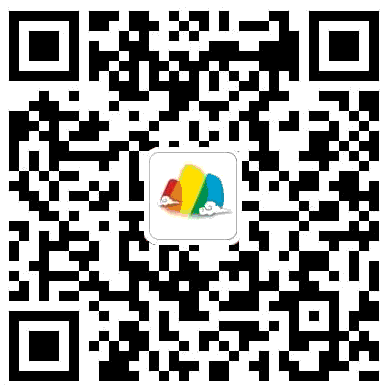# 新人教版小学数学一年级下册期末复习试题（共六套）

2015-05-27 18:08:00

9941

7+13＝          12－6＝       19－8＝       13－7＝      12+5＝

14－6＝         11+0＝        16－5＝       11－3＝      10+5＝

15－9＝         11－5＝       14－7＝       16－6＝      7+8＝

12+4－9＝      10－5+8＝       9+7－2＝          11－5+3＝12-   =6         4+   =13        15-   =8

10+   =10         -5=12          11-    =7

12+   =15       14-   =8         5+2=    - 6五、你知道里面藏着的是“+”还是“-”吗？六、看图列出两道加法算式和两道减法算式。七、填表。八、解决问题。

1阿姨拿了16个，分给小朋友8个，阿姨还剩多少个口答：阿姨还剩（     ）个2、停车场原来有17辆汽车，现在只有9辆，开走了几辆？口答：开走了（     ）辆。

3、15本作业本分给小东和小华，小东分得8本，小华分得多少本？口答：小华分得（     ）本。

4、一共有12个。

（1）左边有5个，右边有几个？（2）花皮球有6个，白皮球有几个？2、在正确答案下面打“√”（4分）学  校 班  级 姓  名 学  号 等  级

8＋6=          58=          129=           159=

5＋7=          116=         105=           113=

14－7=         112=         128=           55=

3＋9=          88=          167=           178=

17－6=         69=          126=           109=

9－8=          137=         133=           168=

7＋7=          115=         66=            125=

6＋4=          107=         1710=          165=

17－9=         137=         148=           159=

10－10=        118=         100=           100=

9＋57=             1888=              1563=

5＋54=             1558=              1029=

7＋84=             1578=              1897=

11－38=            645=               1784=

1、98的和是（        ）。98的差是（        ）。

2、512少（        ），127多（         ）。

3、被减数是14，减数是9，差是（           ）。

4、一个加数是6，另一个加数是8，和是（        ）。

5、54=12－（     =15－（

6、在里填上“＞、＜或＝”。

11－46      4913           4710

15－89       136713        17971

7、看图填算式。(    )＋(    )=(    )       (    )(    )=(    )

(    )－(    )=(    )       (    )(    )=(    )

8、想一想，再填空。

1167=     想：7加（   ）得16167等于（   ）。

2137=     想：10－（    =

3 ＋（    =你会发现，长方形的两条长边的长（    ），两条短边的长（    ）。

 四、数一数，再填空。（5%）五、看图列式计算。（12%）六、用数学。（6%）七、计算.

18-9-4=         15-4-7=          6-7-5=

17-5-8=         16-6-6=          19-10-7=

12-7+8=          15-9+6=           11-4+6=

11-5+9=          5+4+8=             9+2+7=

 14-5= 8+6= 8+30= 16-7= 11-2= 30+5= 20-10= 76+8= 6+4= 72-30= 25+3= 13-6= 25-3= 13-9= 28+2= 28+5= 64+5= 67-60= 8+40= 5+8= 13-4= 12-8= 11-5= 6+5= 70+20= 35+30= 17-8= 20+56= 60-30= 6+36= 14-7= 10+5= 15-9= 9-9= 43-8= 68-50= 48+6= 14-6= 40+20= 24+3= 6+6= 10-7= 6+57= 23-7= 79-6= 13-8= 9+3= 80-6= 35+4= 48-2= 15-7= 53-3= 60-20= 9+25= 100-30= 7+5= 26+5= 12-4= 8+7= 14-3= 45-9= 11-4= 36+4= 7+4= 44+4= 47-5= 27+2= 6+10= 45-7= 10+33= 20+42= 53+4= 18-9= 14-4= 12-9= 34+20= 10-7= 38+7= 20+15= 6+9=

1. 46里面有（     ）个一和（     ）个十。

2. 3个十和8个一合起来是（      ），读作（          ）。

3. 70是（     ）位数，它的两个相邻数是（     ）和（     ）。

4. 53角＝（　 　）角            19角＝（       ）元（       ）角

１元－4角＝（　　 ）角             4角＋9角＝（      ）元（      ）角

4.58元＝（        ）元（        ）角（          ）分

5.  389多（         ），   2062少（         ）， 比284的数是（         ）。

6. 写出4个十位上是3的两位数：                    。（２分）

7. 按规律填一填、画一画。（4分）8. 把下面各数按从大到小的顺序排列。（3分）

67     24       98     42      100      86

）＞      ）＞（     ）＞（    ）＞      ）＞（=
 四、动手操作题。（10分） 1. 看图填一填，涂一涂。（６分）2. 写出钟面上的时间。（４分）_______________         _______________            _______________       _______________1. 直接写出得数。（6分）

34＋209 =    269－3＝     732050

76＋652   2738      568＋7＝

2. 在里填上“>”、“<”或“=”。（6分）

4660＋4       76970          40＋5020＋70

7＋5562       50194          53－453－5

3. 在里填上适当的数。（6分）（1）裙子比毛巾贵多少钱？（３分）

（2）帽子比衣服便宜多少钱？（３分）

（3）衣服和毛巾一共要多少钱？（３分）

3. 卖了16盆鲜花，还剩9盆。（３分）4. 箱子里有24瓶矿泉水。（3分）5. 下面是一（3）班最爱吃的水果的统计图。（7分）

 我们最爱吃的水果（1）完成统计表。（２分（2）爱吃（      ）的人数最多，爱吃（　　　　）的人数最少。（1分）

（3）爱吃西瓜的人数和爱吃梨的人数相差（　　　）人。（１分）

（4）你还能提出什么问题？并解答你所提的问题。（共3分）2分）

7＋9 =         97=         127=

9＋4 =         188=        167=

5＋97=       189+9=        1696=

8＋46=       80+44=        933+9=

9元＋8=     2元－5=      13角＋7=

1．看图填数2．观察下图回答问题：3．看图列式。4．在○里填上“＞”、“＜”或“＝”。

9889        1212       6+813

16－96         60+886          8+4166

5一张50元的人民币可以换（    ）张10元的人民币；一张一元的人民币可以换（   ）张2角的人民币。

6．数一数。1）草莓60个，苹果比它多得多，苹果可能有多少个？2的价钱比30元少一些。一个书包多少元？4＋70 =          129=          905=

74－4=           488=          97 =

60＋9 =          179=          777=13元                  40元

妈妈买了一把尺子和一盏台灯，应付多少元？（5分）25分）1）小小明买一把尺子，给售货员一张10元，应找回多少元？（3分）口答：应找回（      ）元。

2）你还能提什么问题？（提问占2分，解答占3分）口答：（                ）。

1．小雨和小雪共画了15朵花，小雨画了9朵，小雪画了几朵?

2．小明有18枝彩色笔，小刚借走了9枝，小明还有几枝?

3．同学们排队，小兰的前边有5人，后面有7人，这一行共有多少人?

4．小青要练习写16个毛笔字，还剩下8个字没有写，他已经写了几个字?

5．停车场上的汽车开走了6辆，又开走了5辆，一共开走了多少辆?11－8＝         10－2＝        16－7＝        18－10＝

13－5＝         13－9＝        18－9＝        15－6＝

12－7＝         12－8＝        17－8＝        11－2＝

16－9＝         12－3＝        19－9＝        13－4＝

3＋8－9＝       12－5＋8＝     13－8＋6＝     4＋9－7＝

1．（1（22．树上有一些小鸟，先飞走10只，又飞走了6只，两次飞走多少只？3．苹果和梨子一共有14个，其中梨子有6个，苹果有多少个？（1）兔和羊一共有多少只？2）白鸡有4只，黑鸡有多少只？6+7=      5+9=      13-8=       4+6=    3+8=

5+8=      6+8=       13-3=       11-9=     17-9=

12-6=       14-8=      18-9=       12-8=     16-7=

14-5=       15-7=      4+9=      5+7=      6+6=

1.8与7的和是( )87的差是( )

2.两个数的和是14，一个加数是9，另一个加数是( )

3.减数是6，被减数是11，差是( )

4.4比11( )128( )

5.5+2=11-( )=13-( )=15-( )

6.有6根短绳，要连成一根长绳，要打( )个结。

7.姐姐有7妹妹有3姐姐给妹妹( )朵，两人的一样多。

12-8○7   13-65   11-415-8

14-6○8   11-79   17-916-8

2+8○11   13-75   4+89+5

6+(  )=13    7+(  )=11    11-( )=6          5+( )=12

12-( )=7     (  )+8=15    (    )+9=17       7+( )=15

13-( )=5     15-( )=8     16-( )=6          11-(  )=8最后编辑：ppxuan 于 2015-05-28 16:10:53

评论列表
2088
 kitty贝贝 2015/06/04 很不错，又实用，刚好期末复习用 回复
1/1
发表评论
评论通过审核之后才会显示。
分类免费预约体验课